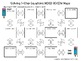# Solving One-Step Equations Mazes and Interactive Notebook FoldablesSubject
Resource Type
Common Core State Standards
Product Rating
File Type

Compressed Zip File

33 MB|34 pages
Share
2 Products in this Bundle
2 products
1. This set of interactive notebook foldables includes many solving one-step equations topics such as:1. Properties of Equalities2. Solving One-Step Equations involving Addition and Subtraction3. Draw a Picture & Write an Equation involving Addition and Subtraction4. Solving One-Step Equations invo
2. There are three mazes included in this product. The first maze includes solving one-step equations that involve addition and subtraction of whole numbers and/or decimals. The second maze includes solving one-step equations that involve multiplication and division of whole numbers and/or decimals.
Bundle Description

This bundle includes my solving one-step equations mazes and my solving one-step equations interactive notebook foldables. Below are the descriptions for each one.

Solving One-Step Equations Mazes:

There are three mazes included in this product. The first maze includes solving one-step equations that involve addition and subtraction of whole numbers and/or decimals. The second maze includes solving one-step equations that involve multiplication and division of whole numbers and/or decimals. There are two versions of the second maze with division problems written first using the division symbol and then in the next maze division is depicted using the fraction bar. The third maze includes solving one-step equations that involve all four operations of whole numbers and/or decimals.

Students solve one-step equations in order to find the correct path from the start to the end. They will complete 9 out of 11 problems if they follow the correct path.

There is also a work space page to give to students to organize their work! I generally copy this on the back of the maze.

This a great activity for a review, independent practice, group work, homework and much more.

Check out more of my maze activities:

Slope Maze

Solving Multi-Step Equations Maze

Solving Consecutive Integers Maze

Solving One-Step Equations Interactive Notebook Foldables:

This set of interactive notebook foldables includes many solving one-step equations topics such as:

1. Properties of Equalities

2. Solving One-Step Equations involving Addition and Subtraction

3. Draw a Picture & Write an Equation involving Addition and Subtraction

4. Solving One-Step Equations involving Multiplication and Division

5. Draw a Picture & Write an Equation involving Multiplication and Division

6. BONUS: Placemat Review

All of these solving one-step equations foldables are designed to fit in a composition notebook if needed or any other notebook you may use and are designed to be an easy print and go for the teacher. They have a few simple cuts that can be cut by the teacher ahead of time or by the students but nothing that is too time consuming. Many of the foldables have lots of practice which you can use in the classroom for guided practice, independent practice or for homework. Foldables are a great way to help students organize their notes and take pride in their notebook!

Check out more of my Interactive Notebook Foldables:

Numeration Interactive Notebook Foldables

Variables, Expressions and Properties Interactive Notebook Foldables

Operations with Decimals Interactive Notebook Foldables

Let's Connect

Blog

Instagram

Email: flip4mathteacher@gmail.com

Total Pages
34 pages
N/A
Teaching Duration
N/A
Report this Resource to TpT
Reported resources will be reviewed by our team. Report this resource to let us know if this resource violates TpT’s content guidelines.